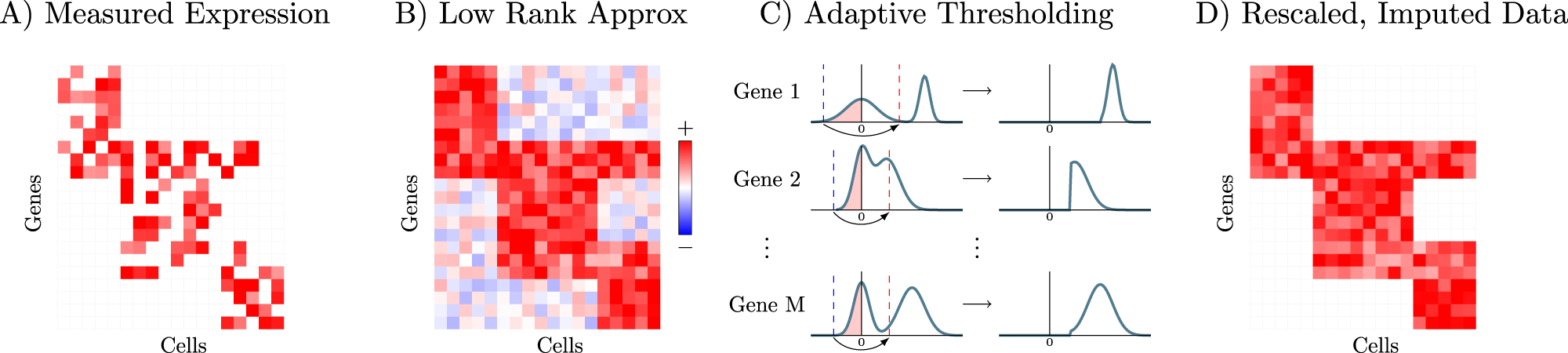# Zero-preserving imputation of single-cell RNA-seq data

A key challenge in analyzing single cell RNA-sequencing data is the large number of false zeros, where genes actually expressed in a given cell are incorrectly measured as unexpressed. Yale University researchers have developed a method based on low-rank matrix approximation which imputes these values while preserving biologically non-expressed genes (true biological zeros) at zero expression levels. The researchers provide theoretical justification for this denoising approach and demonstrate its advantages relative to other methods on simulated and biological datasets.

Overview of the ALRA imputation schemeA Measured expression matrix contains technical zeros (in each block) and biological zeros (outside each block). B Low-rank approximation via SVD results in a matrix where every zero is completed with a non-zero value (i.e., biological zeros are not preserved). C The elements corresponding to biological zeros for each gene are symmetrically distributed around 0, so the magnitude of the most negative elements in each row is also approximately the magnitude of the most positive values assigned to biological zeros. Therefore, thresholding the values in each row, restores almost all of the biological zeros in the imputed matrix (D).

Linderman GC, Zhao J, Roulis M, Bielecki P, Flavell RA, Nadler B, Kluger Y. (2022) Zero-preserving imputation of single-cell RNA-seq data. Nat Commun 13(1):192. [article]